# Beam Bending Final

This is wrapping up, I think.  There’s not much more to do before trying to move on to more practical calculations.  I suppose I could compare numerical large angle results with the small angle analytic results; that’s the main other thing I can think of.  Or maybe I could look at other extensions, like buckling.

Anyway, this post has one more worked example, plus the derivation of the Euler-Bernoulli beam equation from the method I have been using.

Maybe we can start with the derivation first.   Let’s assume we’re looking at a beam between x = a and x = b, and no other boundary conditions are given.

If the force applied between x and x+dx is q(x)dx, then the energy is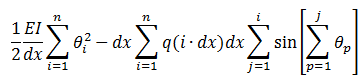(Compare to the energy for a uniformly loaded beam)This equals zero, leading (with just a little bit of re-writing) to the equation: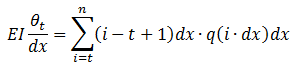What does this equation mean?  We earlier showed that (theta/dx) = f”.  Let’s assume that the index t refers to a point x = c between x = a and x= b.  So, the right side is EI f”(c).

The (i-t+1) dx term must equal (x-c).  (n dx = b, t dx = c — I guess I’m carelessly acting like a = 0, though it doesn’t have to be)  Then the sum is a Riemann sum, so we haveWe could interpret the right side as a sum of “torque densities”.

Differentiate both sides with respect to c gives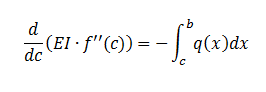Differentiate again andand that is the Euler-Bernoulli equation.

_______________________________________________________________

For the last worked example for now, we’ll consider what Wikipedia calls a “statically indeterminate” case of a cantilever with a uniform load, fixed rigidly at one end and not rigidly at the other:The function to find an extreme of: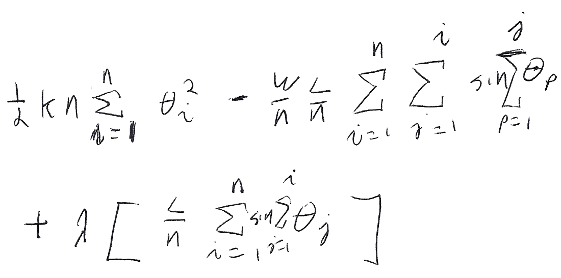The energy is the same as the previous case for a uniformly loaded cantilever. The lagrange multiplier this time is to ensure the height is zero at the other end.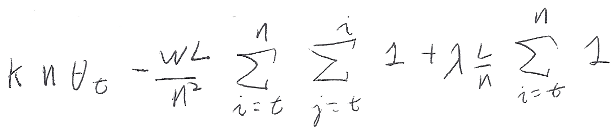Evaluating the sums:The first term (uh, the one starting with WL) is obviously the same as it was for the uniformly loaded case, and since using the small angle approximation means this contributions add linearly, we know that the deflection isThe second term sums towhich we recognize exactly as the form of the deflection for a cantilever with a weight on the end.

Now it all makes sense, even without math.  The Lagrangian multiplier has physical significance as a weight (or force) that is needed to lift the cantilever up to its original position on that side.  And, of course, that’s exactly the force that other support supplies.

Had we thought about this earlier, we probably could have immediately written down the solution as the sum of these two types of solutions.

Anyway, we can plug into the boundary condition, that y = 0 at x = L, to fully solve the problem.The weight is negative because of course the support pushes up.